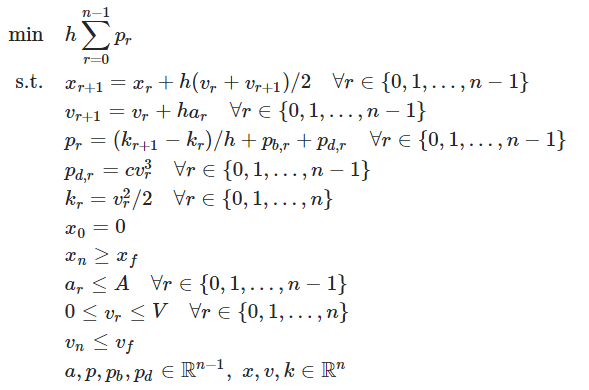# Problem formualtion in CVX- MATLAB, where am I going wrong

Hi, so I am new to this CVX platform and I have to solve this optimization problem,So I have written the code for it and also changed the equalities to make it convex but I think there is some problem in my code which I am not able to figure out,

clc;clear all;
m=10;
x_final = 12;
v_final = 2;
v_max = 10;
a_max = 2;
c_loss = 2;
h = 0.1;
T = 5;
r = T/h;

cvx_begin
variables p(1,r-1) v(1,r) x(1,r) k(1,r) p_d(1,r-1) p_b(1,r-1) a(1,r-1);
% expressions p_b(1,r-1) a(1,r-1);
minimize(h*sum§)
v(1) == 0;
x(1) == 0;
a(:,1) == 2;
v >= 0;
a <= a_max;
v <= v_max;

``````for i=1:r-1
v(i+1) == v(i) + (h*a(i));
x(i+1) == x(i) + ((h/2)*(v(i) + v(i+1)));
k(i) >= (m*(v(i)^2))/2;
p_d(i) >= c_loss*(pow_abs(v(i),3));
k(i+1) == (h*(p(i) - p_b(i) - p_d(i))) + k(i);;
end
x(end) >= x_final;
v(end) <= v_final;
``````

cvx_end

It has been written in MATLAB 2020b, can any point where I am going wrong, this is the output I get when I run it

Trivial infeasibilities detected; solution determined analytically.
Status: Unbounded
Optimal value (cvx_optval): -Inf

Perhaps you made the problem unbounded when you relaxed those equalities to convex inequalities.

Have toy proven the original problem is convex?